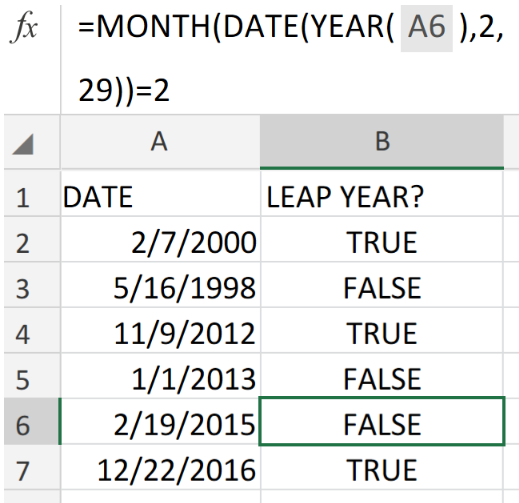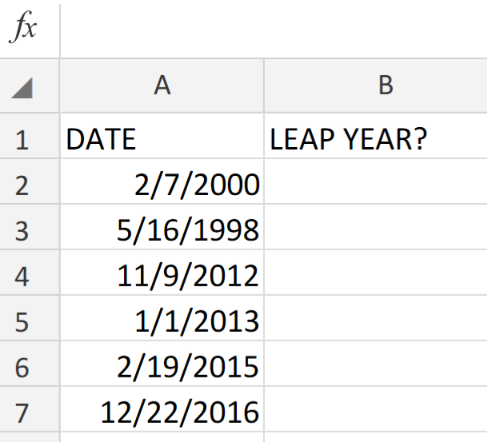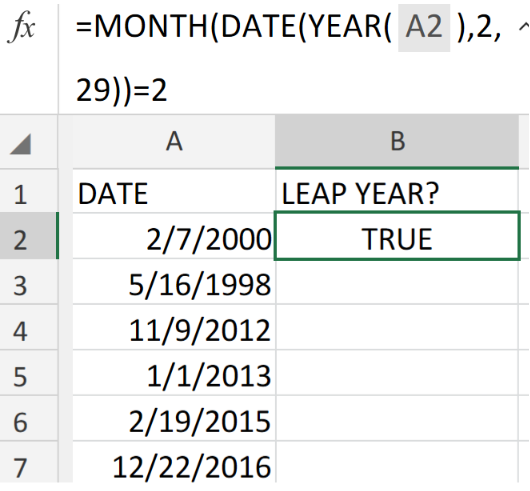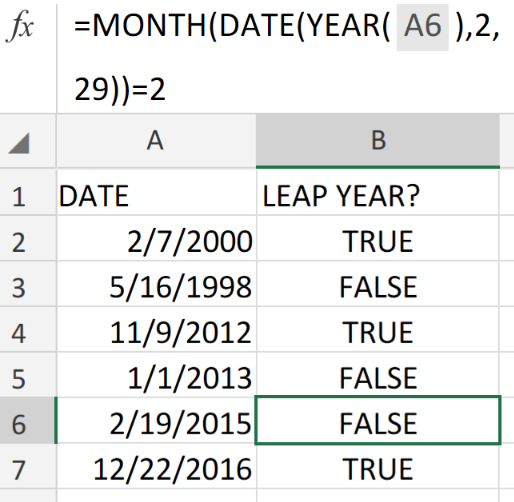Get instant live expert help with Excel or Google Sheets“My Excelchat expert helped me in less than 20 minutes, saving me what would have been 5 hours of work!”

#### Post your problem and you’ll get expert help in seconds.

Your message must be at least 40 characters
Our professional experts are available now. Your privacy is guaranteed.

# How to Check if a Year is a Leap Year in ExcelFigure 1. Year is a Leap Year in Excel.

We are going to use a formula that consists of the Excel MONTH, YEAR, and DATE functions in order to ascertain whether the year of a given date is a leap year,

## Generic Formula

`=MONTH(DATE(YEAR(date),2,29))=2`

In the formula above, February has 29 days during a leap year, and the number 2 is for the month of February.

## How to use the Excel MONTH, YEAR, and DATE Functions.

We are now going to utilize the Excel MONTH YEAR and DATE Functions in 3 simple steps;

1. Enter the day, month, and year values in a column of our worksheet.

Be sure to label a separate column for Excel to indicate which of the dates occur on a leap year.

See example illustrated below;Figure 2. Year is a Leap Year in Excel.

1. In the example illustrated above, we are going to enter the following MONTH, YEAR, and DATE formula into cell B2;

`=MONTH(DATE(YEAR(A2),2,29))=2`Figure 3. Year is a  Leap Year in Excel.

1. After Excel has returned the initial result, copy the formula into the cells down the column for the next results to be returned.Figure 4. Year is a Leap Year in Excel.

If the year is a Leap Year, the formula returns TRUE and if not, the formula returns FALSE.

However, during non-leap years, the date March 1 of the year will be returned by the DATE Function because the 29th day does not exist.

## Instant Connection to an Expert through our Excelchat Service:

Our live Excelchat Service is here for you. We have Excel Experts available 24/7 to answer any Excel questions you may have. Guaranteed connection within 30 seconds and a customized solution for you within 20 minutes

### Did this post not answer your question? Get a solution from connecting with the expert.Another blog reader asked this question today on Excelchat:
Solution examplesUse the Vlookup Function to complete the "employee" column of table 2. Use "job Id" from table 2 as your lookup_value(s) and table 1 as your reference.
Solved by C. H. in 16 minsIf a cell in another sheet is populated I need a vlookup done. If the cell is not populated I need the cell to return blank.
Solved by T. D. in 60 minsI am trying to make a chart that turns a week range red if nothing is entered in the range. If something is entered then I would like it to turn green. Please Help
Solved by E. U. in 43 minsI need a check box to show/hide an answer of an if function
Solved by Z. U. in 23 minsI need a formula that looks up if the date (which looks like "8/1/2018 8:05:36 AM") in Column "C" contains year 2018. And then populates "2018" in Column D, if the date does not contain "2018" then enter False in Column D.
Solved by F. H. in 20 mins## Subscribe to Excelchat.coAnother blog reader asked this question today on Excelchat: